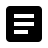## Repetitive statements in C

In programming practices, we may need to execute one or few tasks for more than once. The tasks can be composed of few statements or a function or precedure. These type of tasks are repititive in nature and we write special programming blocks which we are going to discuss here. Let's say we want to add two or more numbers or want to know the average of those numbers. Now we need to add numbers one by one and calculate the final summation.

sum = num1;
sum += num2;

sum = num1;
sum += num2;
sum += num3;

We can have two or three statements to add few numbers but the total count of numbers may be any positive numbers ranging 1-10, 1-100, or more. So basically the number of count is a variable in nature and the program should be flexible enough to deal with this total count. To make it generic we need to alter the programming logic. We can take the numbers in an array and add the array elements till the count is reached

sum = 0;
sum =+ num[i];
Here i can range from 0 to (- 1)

These type of requirement in the programming can be achieved by loop statements.

## Loop statements

In the programming world, repetitive statements can be executed with the help of loop statements. C/C++ and all modern programming languages have basic loop statements to do repetitive statements.

sum = 0;
programming loop (= 0; i < count;) {
sum += num[i];
i++;
}

Writing repetitive statements is easy in C and keywords like for, while, do-while are available for this. Let us discuss these three types of looping statements one by one.

## Repetitive statements with FOR

For loop is a basic loop statement used very often in programming.

for
( <initialization statement>;
<comparison>;
<step statement>)
{
<statement(s)>;
}For loop starts with a one-time initialization statement then a comparison to check whether to continue the loop or exit from it. It also has one step statement which will be executed at the end of each iteration and then it will again go for the comparison. This is good when we are dealing with array and accessing one element at a time in each iteration.
for (= 0; i < array_len; i++)
{
Array [i] = i;
}

## Repetitive statements with WHILE

While loop does not have any initial statement like for loop. It goes straightly to condition checking then executes statements and again comes to condition checking. Thus again it does not have any step statement.
while (<condition statement>)
{
<statement(s)>
}While loop is good when we do not know when to end specifically like linked list iterations.

while (node)
{
node= node->next;
}

## Repetitive statements with DO-WHILE

For do-while has this syntax-

do
{
<statement(s)>;
}
while (<condition statement>);Do-while loop is not different than while loop except for the fact the checking will be done at the end of the loop thus the first iteration goes without any condition checking. Do-while loop is mostly used for the situations where we need to check the condition at the end like a menu driven program where we display entire menu selections and wait for user's input the depending upon user's input we decide whether to stay in the loop of exit from it.
do
{
printf ("To print student's record [p]\n");
printf ("To add new student's record [a]\n");
printf ("To edit existing student's record [e]\n");
printf ("To delete student's record [d]\n");
printf ("To exit [x]\n");
printf ("you select: ");
c = getch();

}
while (!= 'x');
Curly brackets are not needed if there is only one statement inside the loop. C has "continue" and "break" keyword to either continue or exit from the loop. We will discuss more on this in our next section.

You have viewed 1 page out of 248. Your C learning is 0.00% complete. Login to check your learning progress.Write C programming syntax for, while, do while loops.
How to write loop statements in C? How to write a for loop, while loop, do while loop? Study the SYNTAX, FLOW DIAGRAM, with CODE, VIDEO examples.How to convert for a loop to while loop, vice versa?[GUIDE]
Convert for loop to while loop and vice versa. Understand for, while, do-while loops and write code properly. Also understand on how to convert for, while, do-while to other loopsWhen is do-while loop preferred over while loop, Give example?
Understand importance and need of do-while loop. How preferred over while loop, Use of do while over while loop. Give example do while vs while usecaseWrite infinite loop statements for 'while', 'do-while' and 'for'?
What is an infinite loop in programming? Need of infinite loop. Write infinite loop statements in C. Example of infinite loop 'for' 'while', 'do-while'.How to break a loop? How to skip loop iterations with continue? [example and flowchart]
Understanding C continue and break keyword with flowchart and practical example source code. Where to put break or continue in for loop, while loop, do while loop